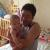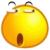## oracle sql 查询 请教个问题

WeirdBIrd 发布于 2013/08/23 18:53

`select so.org_id  from  sys_org so  where so.parent_id`

```where tmp.org_id in(
case when 1= org_choice  then {org_id}
when  2=org_choice then (select so.org_id from sys_org so where so.parent_org_id=org_id)
when 3=org_choice  then (select org_id（界面传递过来的本组织）, so.org_id from sys_org so where so.parent_org_id=org_id)

)```

0oracle 的递归,试试:

```select so.org_id  from  sys_org so
START WITH org_id = 1
CONNECT BY PRIOR org_id = parent_id```

0